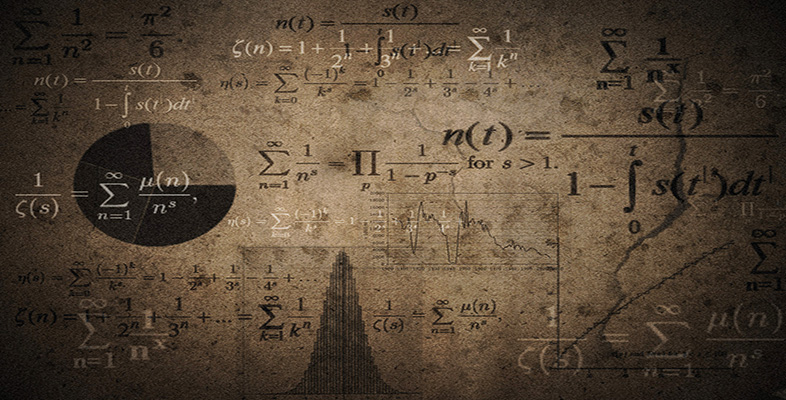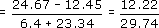Language, notation and formulas

This free course is available to start right now. Review the full course description and key learning outcomes and create an account and enrol if you want a free statement of participation.

Free course

# 2.2 Using the equals sign

As mentioned previously, one of the most misused mathematical symbols is the equals sign, =. It stands for the verb ‘equals’ or the phrase ‘is equal to’ or ‘which equals’, and so it should only come between two things that are equal.

## Example 2

Which of the equals signs should not be included in these calculations?

• (a) 24.67 − 12.45 = 12.22

• (b) 6.4 + 23.34 = 29.74

• (c)The equals signs in (a) and (b) are used correctly, but the first sign in (c) is not. This equals sign is not between two things that are equal, and consequently it should not be there. Also it is at the beginning of a sentence, and when you read it aloud it does not make sense. It would be better replaced by a word like ‘hence’ or ‘so’.

MU120_4M6